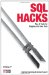# Hack 30. Calculate the Maximum of Two FieldsMAX is an aggregate function; it operates over a single column for several rows. If you need to find the maximum of two fields in the same row you'll need to pull another function out of your toolbox.

Consider a table where each row has two integer values, x and y, as shown in Table 5-8. How can you find the largest of x and y for each id?

Table 5-8. A simple table with x and y values

id x y
A 1 2
B 4 3
C 5 5

If you had the simple two-parameter max function you could just return max(x, y). You can't use the SQL function MAX in this way, but you can use the following formula:

max(x,y) = (x + y + ABS(x y)) / 2

The ABS( ) function calculates the absolute value of a number (the distance from zero to that number). For a positive number, it simply returns the same number unchanged, but it returns the positive size when given a negative input (ABS(-1) = 1). Here's how to use it to calculate the max of x and y:

```mysql> SELECT id, x, y, (x+y+ABS(x-y))/2 FROM t;
+----+---+---+------------------+
| id | x | y | (x+y+ABS(x-y))/2 |
+----+--------------------------+
| A | 1 | 2 | 2.0000 |
| B | 4 | 3 | 4.0000 |
| C | 5 | 5 | 5.0000 |
+----+--------------------------+```

5.7.1. Minimum of Two Values

Should you ever need it, the corresponding definition for minimum works on the principle that adding x + y and then subtracting the distance between x and y is equal to twice the smaller value:

min(x,y) = (x + y ABS(x y)) / 2

5.7.2. Alternative Functions

In Oracle and MySQL you can also use the GREATEST or LEAST function to do the same job. And in any database, the expression CASE WHEN x>y THEN x ELSE y END is equivalent.

5.7.3. Hacking the Hack

If you need to find the maximum of three fields you can find max(x,max(y,z)) and use the same formula, but now the expression starts to get uncomfortably large. However, you can use a derived table with three rows for every row in t. The outer SELECT can find the MAX:

```SELECT id,MAX(m) FROM
(SELECT id,x AS m FROM t
UNION
SELECT id,y FROM t
UNION
SELECT id,z FROM t) u
GROUP BY id```

In SQL Server and PostgreSQL, you can form a UNION containing x, y, and z on the SELECT line; you can take the MAX of this with the standard aggregating function:

```scott=> SELECT x, y, z,
scott-> (SELECT MAX(m) FROM
scott(> (SELECT x UNION SELECT y UNION SELECT z) AS u(m)) AS theMax
scott-> FROM t;
x | y | z | themax
---+---+---+--------
1 | 2 | 3 | 3
4 | 3 | 2 | 4
5 | 5 | 5 | 5```

The corresponding code does not work in MySQL or Oracle. Even though x, y, and z are in scope the parser does not recognize them.SQL Hacks
ISBN: 0596527993
EAN: 2147483647
Year: 2004
Pages: 147